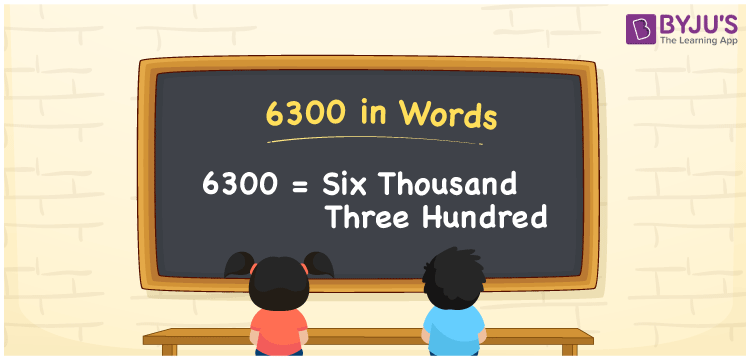# 6300 in Words

In English, 6300 is written as Six Thousand Three Hundred. We know that number names are represented using ones, tens, hundreds, thousands places of the number. Hence, a place value chart helps a lot to write numbers in words. If you paid Rs. 6300 for a water heater, you can write, “I paid Rupees Six Thousand Three Hundred for a water heater”. 6300 is a cardinal number as it represents a certain amount. Let us learn how to write and read the number 6300 in words here in detail.

 6300 in words Six Thousand Three Hundred Six Thousand Three Hundred in numerical form 6300

## 6300 in English Words

Generally, we write numbers in words in English as it is a widely used language in the Education field. Hence, 6300 in words is written as Six Thousand Three Hundred.## How to Write 6300 in Words?

Find the place value of each digit in 6300 while writing the number name. The below chart denotes the place value chart for the number 6300.

 Thousands Hundreds Tens Ones 6 3 0 0

Therefore, the expanded form can be written as:

6 x Thousand + 3 x Hundred + 0 × Ten + 0 × One

= 6 x 1000 + 3 x 100 + 0 x 10 + 0 x 1

= 6000 + 300 + 0 + 0

= 6000 + 300

= 6300

= Six Thousand Three Hundred

Hence, 6300 in words is written as Six Thousand Three Hundred

Interesting way of writing 6300 in words

6 = Six

63 = Sixty-Three

630 = Six Hundred and Thirty

6300 = Six Thousand Three Hundred

Thus, the word form of the number 6300 is Six Thousand Three Hundred

6300 is a natural number that is one less than 6301 and one higher than 6299

• 6300 in words – Six Thousand Three Hundred
• Is 6300 an odd number? – No
• Is 6300 an even number? – Yes
• Is 6300 a perfect square number? – No
• Is 6300 a perfect cube number? – No
• Is 6300 a prime number? – No
• Is 6300 a composite number? – Yes

## Frequently Asked Questions on 6300 in Words

Q1

### Write 6300 in words.

6300 in words is written as Six Thousand Three Hundred.
Q2

### Simplify 6000 + 300 and express in words.

Simplifying 6000 + 300, we get 6300. Hence, 6300 in words is Six Thousand Three Hundred.
Q3

### Is 6300 an odd number?

No, 6300 is not an odd number.